View Course Path

# Fault Modeling in Chip Design – VLSI (DFT)

Contents

## What is Fault Modeling?

Fault Models aren’t only specific to Design for Testability. Fault models are used in almost all branches of engineering.

A Fault Model is an engineering representation of something that could go wrong in the production, development, or operation of a piece of equipment or product. From this model, the designer or user can then efficiently and effortlessly predict the consequences of this particular fault.

A conceivable approach to testing would be to keep a database of all the faults that are observed over time and then check each chip against each fault in the database. But this is not possible because 1) It would take too much time 2) New faults are being discovered every day. Hence, instead of cataloging the faults, we catalog the behavior that arises out of these faults. This number is smaller and we can just test for these abnormal behaviors and then identify the fault. This is the essence of fault modeling.

## Why Fault Model?

The number of physical defects in a chip can be too many. A modern VLSI chip can contain millions of transistors. It is very challenging (next to impossible) to count and analyze all possible faults. Hence, we abstract physical defects and define some logical fault models.

• Drastically reduces the number of faults to be considered.
• Makes test generation and fault simulation possible.
• We can evaluate fault coverage and compare test sets.

## Levels of Abstraction

A VLSI chip has various levels of abstraction.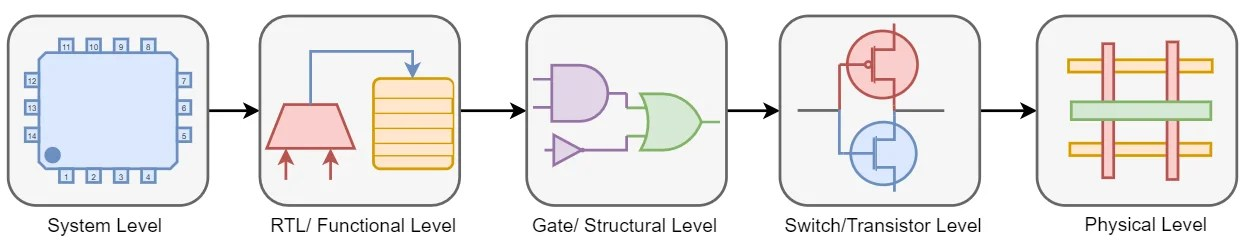### Physical Level

As we move towards the extreme right (lowest abstraction), the fault model becomes more accurate, but the number of possible faults will also increase. As you can figure out, the number of transistors will be larger than functional blocks; hence fault modeling becomes much more difficult.

Higher-order faults become more localized at Physical Level. These higher-order faults are equivalent to each other. For example, a short of source and drain of an nMOS is just equivalent to its gate terminal stuck-at-zero (don’t worry if you didn’t get it, you will understand this very soon). The point is that Physical-Level abstractions increase the number of faults to be considered, thereby leading to more complexities. Hence, fault representation at this level becomes a hectic task.

Let’s see how a manufacturing defect can cause a fault in a circuit. Below is the Physical Level diagram of a CMOS inverter.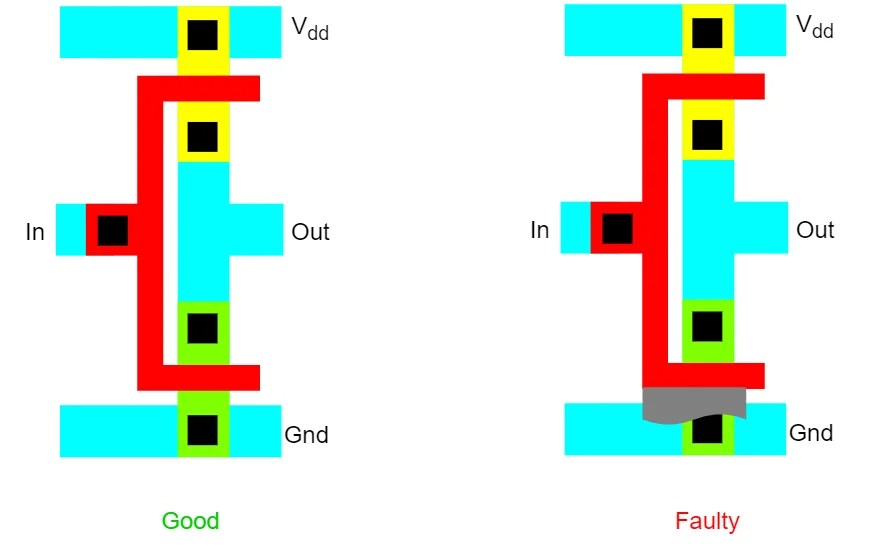Due to a manufacturing process problem in the lithographic process, not all of the metal shown in red is etched away properly, leaving an excess of metal at the bottom of the shape shown in grey. This excess of metal overlays the pull-down nMOS gate with the drain, which is connected to the ground power distribution trace. So, the transistor gate will always be shorted to the ground. This is a defect. This defect can be represented by a fault model. In this case, it is a stuck-at-0 fault (as the name suggests) at the gate terminal. We will study stuck-at-faults in detail in later sections. Consequently, the transistor output will always be stuck-at-1 and can be modeled by the same. This fault may cause abnormal behavior to the output response of the chip. This is known as a failure in the chip.

Faults at these levels are technology-dependent. Hence, a fault model at this level can’t be applied to other technologies. (Faults in TTL technology won’t necessarily translate to CMOS technology). In addition to that, the physical backend layout doesn’t feel comfortable in our eyes too.

### System Level

As we move towards the extreme left (highest abstraction), the fault modeling becomes much more comfortable. And that’s because we need to take care of fewer things. But this does not bode well for accuracy.

For example, assume a fault at just one location of an 8K memory. In Physical Level, our job is to locate the type of fault and the specific location out of all 8194 locations. But in System-Level, we can just discard the whole chip and consider it to be faulty. Hence, higher-level abstraction does not provide much information about the origin or type of fault, so this type of modeling is not prevalent in the industry.

Hence, we can’t use an extreme level of abstraction (far-left: System-Level or far-right: Physical-Level) to model or simulate faults in digital circuits. We need to be somewhere in the middle. Therefore, the faults are generally modeled at Gate Level, Switch Level, and Functional Level. In most of the parts in this DFT course, we will be focusing on particularly these three abstractions to model our faults.

### Functional Level

RTL or Functional Level modeling proves very efficient in certain types of blocks. And the tests for checking the faults can be generated directly with minimal effort. But the main disadvantage is that faults are block-specific, not universal. For example, we can’t apply the same testbench to test different functional blocks like a multiplexer, decoder, memory, etc. Each of these elements needs different test sets.
Moreover, faults may not have any apparent correlation to the physical defects. Take the previous example in the physical level; the stuck-at faults pretty much signifies that the circuit must have its nMOS transistor shorted to ground. There is only one possible defect corresponding to this fault. But after modeling fault at such a higher abstraction (at Functional level), it becomes complicated to locate the actual cause of the defect. There are so many possible defects to choose from.

#### Memory Fault Model

However, one place where functional abstraction seems superior is the memory fault model. Memories are fundamental parts of digital system design. Each memory cells in each memory location have a substantial amount of similarity among them. Hence, a particular category of fault model is specially reserved for memories. These are called memory fault models. We will cover the memory fault model in more detail in the Memory Testing part of this course.
Memory Fault Models - Types
• Memory Stuck-at Faults
• Memory Transition Faults
• Memory Coupling Faults:
1. Inversion
2. Idempotent
3. K-cell coupling

### Structural/Gate Level Fault Model

At Structural Level, the circuit is specified as a schematic, typically at the level of gates and flip-flops. There are a few assumptions:

• The blocks (e.g., gates) are fault-free.
• The interconnections between blocks can be faulty.

The basic idea is to ensure that the interconnections are fault-free, and can carry both logic-0 and logic-1 signals. Popular Structural Level fault models are:

1. Stuck-at fault model
2. Bridging fault model
3. Delay fault model

#### Stuck-at Faults

The most common model used for logical faults is the single stuck-at fault. Here we assume that some of the circuit lines are permanently fixed at logic-0 or logic-1 due to some failures.

Stuck-at-0 and stuck-at-1 faults are often abbreviated to s-a-0 and s-a-1, respectively. Let’s observe how these faults appear in the circuit. Below is the CMOS representation of logic,$Z=\overline { (A+B)\cdot (C+D)\cdot E\cdot F }$Now, say, due to some unfortunate circumstances, our handsome circuit got in some trouble. The source and drain of M2 MOS are short. This short can be modeled as a stuck-at-1 fault at input E, as both of these conditions will exhibit the same behavior. A stuck-at-1 fault at input will eventually force M2 MOS in the saturation region (or short-circuit).

Likewise, the source of M1 MOS is disconnected from the ground (remains open). The equivalent model of this condition is a stuck-at-0 fault at input F.

Due to the presence of these faults, the circuit will misbehave and will cause a failure in the system.

The single stuck-at fault model is often referred to as the classical fault model and offers a good representation for the most common types of defects like shorts and opens in metal oxide semiconductor (CMOS) technology.

But this is not a good abstraction level to represent the faults. Instead, we use Gate Level modeling to represent faults in DFT. Let’s level-up the abstraction!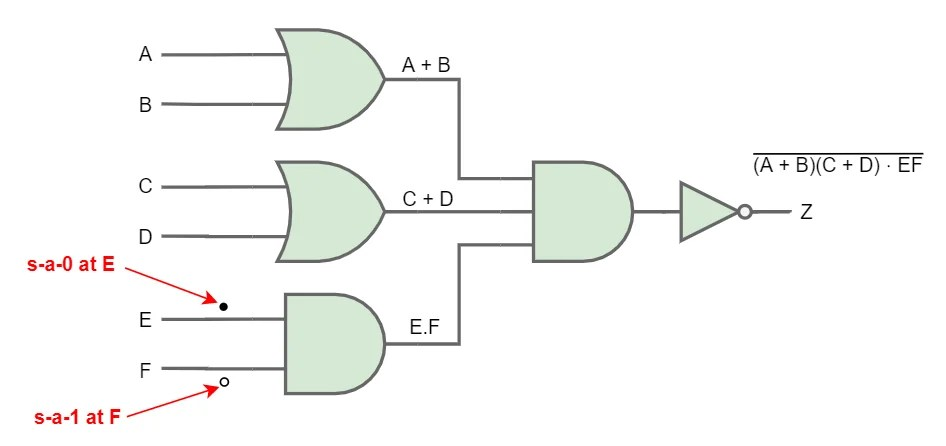In this course, at the gate-level schematic, we will symbolize stuck-at-0 fault as • and stuck-at-1 fault as o.

Note that stuck-at faults are all manifestations of physical faults. It doesn’t mean that physically the wires are faulty. There may be some fault inside a gate (or transistor) that leads to the wire behaving as if it is stuck-at some logic value.

In the previous example, we only considered two faults examples. But in this circuit, there may be numerous examples of stuck-at faults. To symbolize all of them, we need to assign each net by • and o symbol. The following figure indicates that any of the nets (or transistors) are equally susceptible to stuck-at faults. This doesn’t mean these wires are actually faulty; this is just a representation of possible fault areas in the circuit, which can be analyzed using this model.Stuck-at faults can be further classified into again two parts:

##### Single Stuck-at faults

Only one wire of the circuit has a stuck-at fault at any given time. This is the most widely used fault model in the industry.

For a circuit with “n” wires, the total number of single stuck-at faults is “2n”.

For the previous example, since no. of wires is 11; hence we got 22 total stuck at faults (11 s-a-0 + 11 s-a-1).

##### Multiple Stuck-at faults

Any no. of circuit wires can have stuck-at faults at any given time.

Since there are three total classifications for every wire (good, s-a-1 faulty, s-a-0 faulty), therefore, for a circuit with “n” wires, the total number of multiple stuck-at faults is “3n -1″.

For the previous example, there would be${ 3 }^{ 11 }-1=117,146$ multiple stuck-at faults for just a small circuit.

Hence, to simply our test pattern, we only consider single stuck-at faults. It is a good approximation. According to research, a test that detects all single stuck-at faults also identifies a large percentage of multiple stuck-at faults (> 95%).

The main advantage of this fault is that it is technology independent.

#### Bridging Faults

A bridging fault is said to have occurred when two or more signal lines in a circuit are accidentally connected together. It is quite possible due to imperfection during layout fabrication.

If an element is short to power (VDD) or ground (VSS), it is equivalent to the stuck-at fault model that we just studied.

Bridging faults at the gate level have been classified into two types: input bridging and feedback bridging.

• An input bridging fault corresponds to the shorting of a certain number of primary input lines. A feedback bridging fault results if there is a short between an output and an input line.
• A feedback bridging fault may cause a circuit to oscillate, or it may convert it into a sequential circuit.

Bridging faults in a transistor-level circuit may occur between the terminals of a transistor or between two or more signal lines. These can be further classified into two types: wired-AND bridging and wired-OR bridging. Below is a simple gate-level representation of 2:1 multiplexer. In the faulty circuit, two nets are short by a red curved line.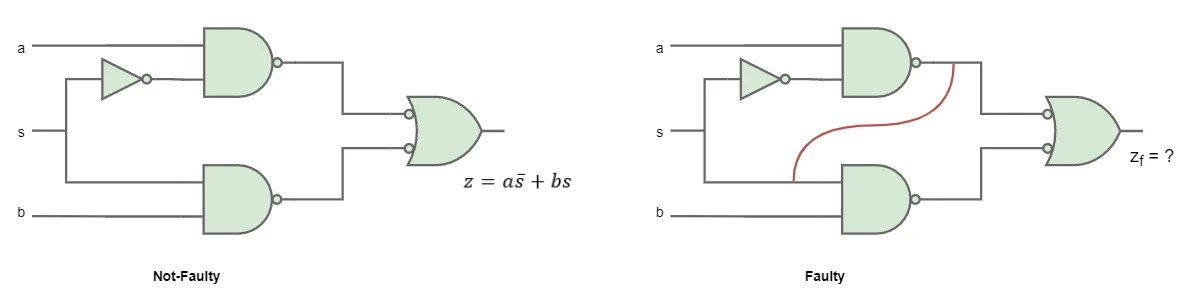How will the output change? Well, this can be modeled by bridging faults, as discussed, there are two different ways to model this. These modeling techniques depend on the technology (e.g., TTL, CMOS) in which the logic is implemented.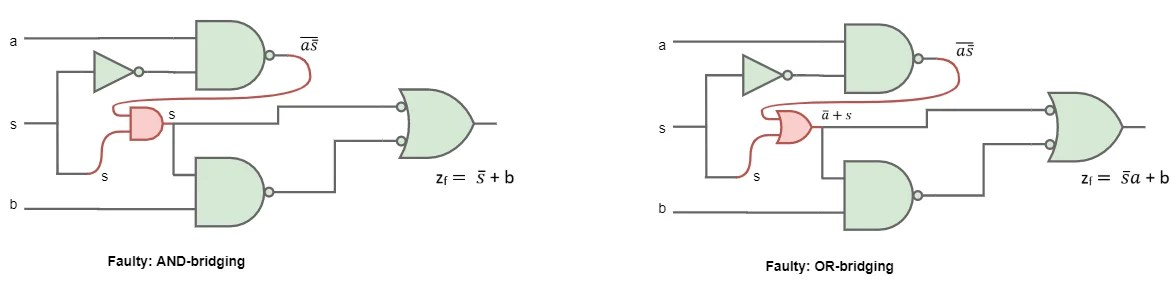Note that z (faulty) or${ Z }_{ f }$ can be different for both these models.

#### Delay Faults

Delay faults are those in which a pin of a gate responds to the transition too slowly on a particular set of stimuli, plus a specific transition.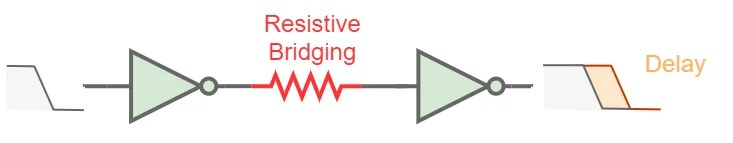• Systematic defects: Crosstalk, process variation in${ V }_{ t }$. E.g., if we have a rising transition and falling transition in two wires very close to each other. The capacitive coupling between them may result in a slower transition to the output.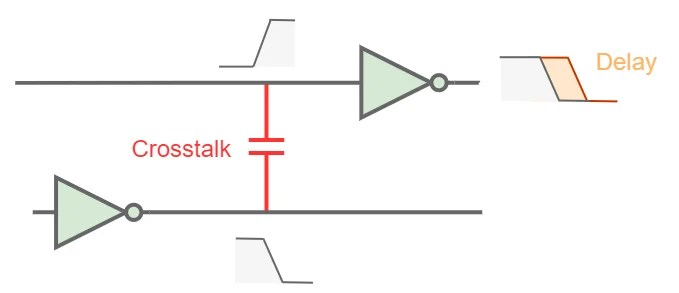Note that, delay faults change circuit timing but not the functionality. A delay fault causes a circuit to fail at a specified speed but may produce correct output at a slower speed.

As opposed to stuck-at faults, delay fault requires a two-pattern test or a set of two test vectors.

• V1: initialize circuit state; apply 00 test vector to the OR gate.
• V2: launch transition, propagate fault effect to output; apply 01 test vector to the OR gate.The actual delay time is calculated by considering the propagation delay of the OR gate. The worst-case delay ‘T’ is set by the tester, and the test pattern is applied. ‘V2’ vector turns the output to logic-1. If the output is shown before the stipulated time ‘T,’ the test is passed, otherwise failed.

But how do we model this fault? There are two main delay fault models:

• Path delay fault model (PDF)
• Transition delay fault model (TDF)

Let’s understand these fault models with an example. Following is a circuit with cascaded AND gates. The propagation delay for a good AND gate is 2.0 ns. Let’s say this circuit is operated at 100 MHz (10 ns clock period). In good condition, this circuit would work perfectly as the maximum delay contributed by the AND gates is 4 x 2.0 ns = 8.0 ns.

##### Path delay fault model

Let’s say due to some faults in gates propagation delay of each gate is increased by some amount. These modified delays are indicated in the diagram in orange color. Will this affect the behavior of the circuit?Let’s calculate the propagation delay (PD) to output X and Y.

PD for output X = 3.0 + 2.6 + 2.7 = 9.2 ns < 10 ns (pass)

PD for output Y = 3.0 + 2.6 + 2.7 + 2.9 = 12.1 ns > 10 ns (fail)

The PD for output X is well under limits, but PD for output Y exceeds the clock period, which is highly undesirable for timing analysis.

This circuit has nine paths from primary inputs to primary outputs. These are AFGHX, BHGHX, CGHX, DHX, AFGIY, BFGIY, CGIY, DIY, EY. For each path in a circuit, there are two potential path delay faults or polarity: falling polarity and rising polarity. So, there are 9 x 2 = 18 path delay faults in the circuit. In the previous example, we determined the rising polarity fault for path AFGHX and AFGIY. The same analysis can be done for falling polarity too.

No. of path delay faults in a circuit = 2 x No. of paths
##### Transition delay fault model

In this model, faults are assumed to be lumped in a single node, as shown by a red cross. Each node has two transition delay faults: slow-to-rise (STR) and slow-to-fall (STF). Let’s analyze the previous example using TDF.

Lumped delay = Extra delay caused by the defect

= PD for faulty circuit to farthest primary output – PD for good circuit to farthest primary output

= 12.1 – 8.0 = 4.1 ns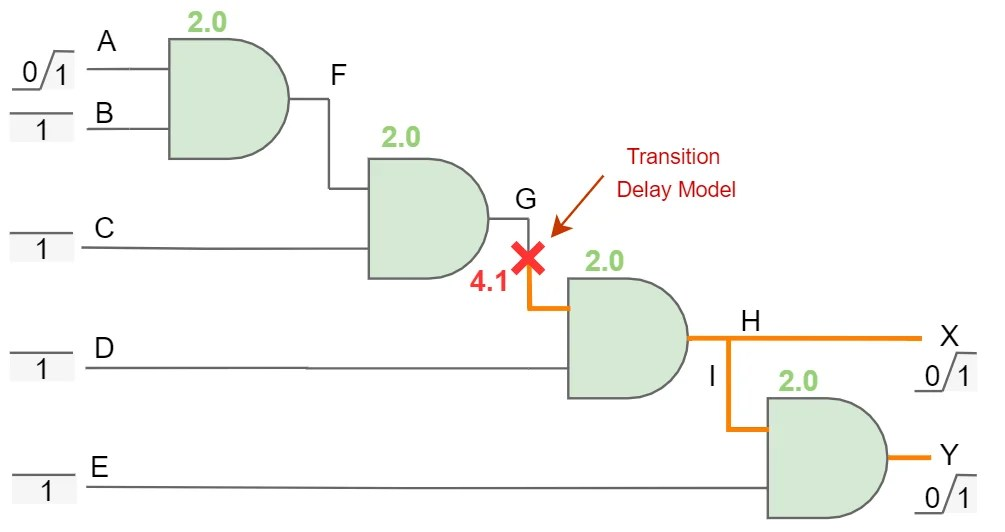PD for output X = 2.0 + 2.0 + 4.1 + 2.0 = 10.1 ns < 10 ns (fail)

PD for output Y = 2.0 + 2.0 + 4.1 + 2.0 + 2.0 = 12.1 ns > 10 ns (fail)

But due to this assumption, even output X fails, but for the real case, it should not. We analyzed TDF for STR. The same analysis can be done for STF too.

No. of path delay faults in a circuit = 1-STR + 1-STF = 2

This does a pretty good job in simplifying the fault model though.

Difference between Path delay fault model and Transition delay fault model
 Path delay fault model Transition delay fault model Distributed along the paths. Lumped in a single node. Two faults per path: Rising polarity and Falling polarity. Two faults per node: slow-to-rise and slow-to-fall. In the worst case, no. of path delay faults can be exponential to the no. of gates. Not scalable for large circuits. High complexity. No. of faults is always 2. Hence it is linear to the no. of gates. Easily scalable for large circuits. Small fault size hence more accurate. Large fault size hence less accurate.
Delay Faults passes Stuck-at tests. Since s-a-0 and s-a-1 faults are just an extreme of a slow-to-rise and slow-to-fall faults respectively, so transition delay faults can be considered as a superset of stuck-at faults. It means if we test a circuit for transition delay faults, the stuck-at faults get automatically tested. Hence, the delay fault model is much better and superior to stuck-at faults.

### Switch Level Fault Model

Here, the circuit is specified at the transistor level. For example, a netlist of CMOS gates. MOS transistors are considered as ideal switches in this model. Two types of switch level fault models are common:

• Stuck-Open Fault
• Stuck-Short Fault

#### Stuck-Open Fault Model

In this fault type, a transistor becomes permanently non-conducting due to some defect. The gate output may depend on its previous state. We can say that the circuit exhibits sequential behavior. It would typically require two test vectors that are to be applied in sequence.

Let’s take the example of a 2-input NOR gate in CMOS.The load Capacitance CL is shown. MOS M1 is stuck open, as shown in the figure, which means Vdd is disconnected from the CMOS logic. Due to the fault, no current flow is allowed in pull-up logic. Hence, we must apply a test vector that must result in the flow of current in pull-up logic (in the non-faulty circuit). Only this test will differentiate between the results in faulty and non-faulty operations so that we could examine the output and decide whether this fault has occurred or not.

The only test vector possible is AB = 00. This input makes both M1 and M2 conducting, and Vdd is connected to the output. Pull-up logic will charge the load capacitor, and we will observe logic-1 at output if the circuit is not faulty. But since it is defective, it will not be connected to Vdd. Will it show logic-0 then? No, neither the pull-up network or the pull-down network is active. So, F will be floating or in high impedance. It may show logic-0 or logic-1, depending upon its previous value. Our simple combinational logic is now showing dynamic sequential behavior.

To counter this problem, we additionally apply an initial test vector AB = 10 to first discharge the load into a known state logic-0. So, we need to apply a pair of vectors in a particular sequence. In this case, the test pattern is AB = {10, 00}. This experiment is also known as the Two-pattern test.

#### Stuck-Short Fault Model

Here a transistor is permanently conducting (i.e., source-drain shorted) in the presence of a fault.

In this case, checking the logic value at the output may not be sufficient. Both pull-up (pMOS) and pull-down (nMOS) networks may become conducting. This condition behaves like a voltage divider (depending on ON-resistance of pull-up and pull-down network), thereby causing the output to reach some indeterminate level.

Due to this, high current will be flowing from Vdd to GND. It will eventually increase the static power dissipation. Ideally, CMOS logic considerably consumes zero static power. Hence, we can detect this type of fault by measuring static power dissipation.  Test vector will be such that it causes a conducting path from Vdd to GND in the presence of a fault.We apply test vector AB = 10. In a non-faulty circuit, this should turn off the pull-up logic. But the fault will cause the pull-up logic to turn on, resulting in a heavy static current.

##### IDDQ Testing

The above experiment is also known as IDDQ testing (Quiescent Drain Current testing).

Advantage: Apart from stuck-short faults, this testing has high defect coverage for other faults too (including stuck-open as well as bridging faults).

Disadvantage: IDDQ testing is losing relevance in deep sub-micron CMOS technology, as the transistor leakage currents become comparable with the IDDQ current. Moreover, there is a design constraint that the circuit must be designed with low IDDQ.

No. of possible stuck-open/short faults in a circuit = No. of transistors in Switch level abstraction

## Summary

In this section, we saw how physical defects occur in VLSI circuits and the proper way to represent those defects using fault modeling techniques. We came across various levels of abstractions. We also learned to generate test vectors for determining stuck-open/short faults. In the next section, we’ll explore more test generation procedures as well as examples.

The overall conclusion is that fault modeling makes our life more comfortable in the testing of VLSI circuits. Although in the industry, we won’t test all the transistors by ourselves one-by-one. Automated software does these. These fault models prove efficient in the design of computer programs for detecting faults in VLSI CAD tools. The DFT engineer just needs to command these CAD tools using some scripting languages.

Related courses for this will be up soon!

This site uses Akismet to reduce spam. Learn how your comment data is processed.

Top